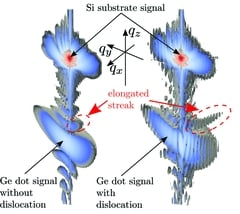•# Algorithms for the calculation of X-ray diffraction patterns from finite element data

## J. Appl. Cryst. (2010). 43, 1287-1299 (doi.org/10.1107/S0021889810032802)Simulated 3D intensity distribution of a coherent and a dislocated quantum dot computed with one of the developed algorithms.

The use of CCD detectors in diffraction experiments allows the collection of 3D data sets containing much more information than scans in 1 or 2 dimensions. This, however, dramatically increases the demands on data analysis, which is based on a simulation of the scattered intensity from finite element modeling of the atomic displacements in a sample. We developed algorithms for the efficient calculation of such 3D diffraction data. As application examples the quantitative interpretation of diffraction data from dislocation arrays and quantum dots is presented.

E. Wintersberger, D. Kriegner, N. Hrauda, J. Stangl and G. Bauer